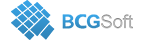BCGSuite for MFC
 void CBCGPChartMAFormula::SetMAType ( MovingAverageType maType, BOOL bGenerateDataPoints = `TRUE` )

Sets moving average type.

Call this method to change the moving average type. If the specified type differs from the current, the data points of output series will be recalculated automatically.

maType can be one of the following enumerated values:

• MA_SIMPLE,
• MA_EXPONENTIAL,
• MA_SMOOTHED,
• MA_LINEAR_WEIGHTED
Parameters
 maType Specifies the new moving average type. bGenerateDataPoints TRUE - recalculate output data points immediately.